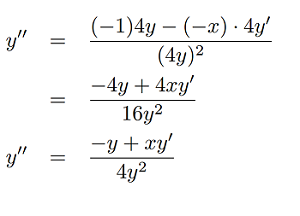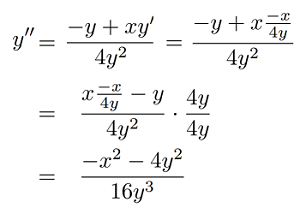# Second Derivative: Test, Examples

Contents:

## What is a Second Derivative?

The second derivative (f′′), is the derivative of the derivative (f). In other words, in order to find it, take the derivative twice.

One reason to find a 2nd derivative is to find acceleration from a position function; the first derivative of position is velocity and the second is acceleration. This is useful when it comes to classifying relative extreme values; if you can take the derivative of a function twice you can determine if a graph of your original function is concave up, concave down, or a point of inflection.

## Examples

Example question 1: Find the 2nd derivative of 2x3.

Step 1: Take the derivative:
f′ 2x3 = 6x2
f′ 6x2 = 12x

Example question 2: Find the 2nd derivative of 3x5 – 5x3 + 3

Step 1: Take the derivative:
f′ 3x5 – 5x3 + 3 = 15x4 – 15x2 = 15x2 (x-1)(x+1)
f′ 15x2 (x – 1)(x + 1) = 60x3 – 30x = 30x(2x2 – 1)

That’s it!

Note: You can’t always take the second derivative of a function. For example, the derivative of 5 is 0.

## Find the Second Derivative Implicitly

Like the “usual” way of finding second derivatives, finding the second derivative implicitly involves two steps: implicitly differentiating twice.

The key to finding the second derivative implicitly requires a good understanding of the chain rule. For example, to take the derivative of an expression like 4y2 with respect to x, you have an inside function and an outside function:

1. Take the derivative with respect to y,
2. Multiply by y′.

That results in 8yy′.

## Finding the Second Derivative Implicitly: Example

Example question: Find the second derivative implicitly of x2 + 4y2 = 1.

## Part One: Finding the First Derivative Implicitly

Step 1: Take the derivative(s) of the left hand side. We have two parts to differentiate: x2 and 4y2:

• x2 (using the power rule) =
2x
• 4y2:
1. Take the derivative with respect to y,
2. Multiply by y′.

Solution: 8yy′.

Combining the answers (because of the Sum Rule) we get:
2x + 8yy

Step 2: Take the derivative of the right hand side. The derivative of a constant is 0.

Step 3: Place your answers from the left hand side (Step 1) and right hand side (Step 2) back together:

2x + 8yy′ = 0

This is the first implicit derivative.

You may be wondering why I split this up into three parts. After all, finding the derivative of 25 is something you can probably do in an instant. That’s because some implicit derivatives are more complicated (e.g. 3xy2 – 2x = 4y) and breaking the problems up into small chunks can make it easier to keep track of the parts.

## Part Two: Finding the Second Derivative Implicitly

You could jump right in at this point and re-run the steps above to get the second derivative. However, you’ll end up with yy′′ in the solution, which means you’ll have to backtrack and solve the earlier equation for y′.
Rather than do that, I like the approach given on MIT’s Open Courseware site : It’s better to streamline and solve for y′ first.

Step 3: Solve for y′:

• 2x + 8yy′ = 0
• 8yy′ = – 2x
• y′ = – 2x/8y
• y′ = – x/4y

Step 4: Differentiate both sides again. The right hand side of this particular equation is solved with the quotient rule:Step 5: Substitute –x/4y for y(Step 3):Step 6: Substitute in the original equation x2 + 4y2 = 1. (Note: A popular online calculator skipped this step!):

Solution: y′′ = -(1 / 16y3).

## Second Derivative Test

This test is used to find intervals where a function has a relative maxima and minima. You can also use the test to determine concavity.

## The second derivative test for extrema

The test for extrema uses critical numbers to state that:

• If the 2nd derivative f′′ at a critical value is positive, the function has a relative minimum at that critical value.
• If the 2nd derivative f′′ at a critical value is negative, the function has a relative maximum at that critical value.
• If the 2nd derivative f′′ at a critical value is inconclusive the function may be a point of inflection.

## Test for concavity

The second derivative test for concavity states that:

• If the 2nd derivative is greater than zero, then the graph of the function is concave up.
• If the 2nd derivative is less than zero, then the graph of the function is concave down.Inflection points indicate a change in concavity. Photo courtesy of UIC.

Example problem: What concavity does the graph x3 have between -2 and 3? Where are the local minimum(s) and local maximum(s)?

Step 1: Find the critical values for the function.

1. Take the derivative: f′= 3x2 – 6x + 1.
2. Set the derivative equal to zero: 0 = 3x2 – 6x + 1.
3. Solve for the critical values, using algebra.

There are two critical values for this function:
C1:1 – 13√6 ≈ 0.18.
C2:1 + 13√6 ≈ 1.82.

Step 2: Take the second derivative (in other words, take the derivative of the derivative):
f′ = 3x2 – 6x + 1
f′′ = 6x – 6 = 6(x – 1).

Step 3: Insert both critical values into the second derivative:
C1: 6(1 – 13√6 – 1) ≈ -4.89
C2: 6(1 + 13√6 – 1) ≈ 4.89.

The second derivative at C1 is negative (-4.89), so according to the second derivative rules there is a local maximum at that point.
The second derivative at C1 is positive (4.89), so according to the second derivative rules there is a local minimum at that point.Step 4: Use the second derivative test for concavity to determine where the graph is concave up and where it is concave down. For this function, the graph has negative values for the second derivative to the left of the inflection point, indicating that the graph is concave down. The graph has positive x-values to the right of the inflection point, indicating that the graph is concave up.The above graph shows x3 – 3x2 + x – 2 (red) and the graph of the second derivative of the graph, f′′ = 6(x – 1) green. Positive x-values to the right of the inflection point and negative x-values to the left of the inflection point.

## Only Critical Point in Town TestGraph showing Global Extrema (also called Absolute Extrema) and Local Extrema (a.k.a. Relative Extrema).
The Only Critical Point in Town test is a way to find absolute extrema for functions of one variable. The test fails for functions of two variables (Wagon, 2010), which makes it impractical for most uses in calculus. Most mentions of the test in the literature (most notably, Rosenholtz & Smylie, 1995, who coined the phrase) show examples of how the test fails, rather than how it works.

## Definition for the Only Critical Point in Town test

The test states that:

Suppose that a continuous function f, defined on a certain interval, has a local extrema at point x0. If x0 is the function’s only critical point, then the function has an absolute extremum at x0

To put that another way, If a real-valued, single variable function f(x) has just one critical point and that point is also a local maximum, then the function has its global maximum at that point (Wagon 2010).

## Definition by Derivatives

The only critical point in town test can also be defined in terms of derivatives:

Suppose f : ℝ → ℝ has two continuous derivatives, has a single critical point x0 and the second derivative f′′ x0 < 0. Then the function achieves a global maximum at x0: f(x) ≤ f(x0)for all x ∈ &Ropf.

The test is practically the same as the second-derivative test for absolute extreme values. The second-derivative test can be used to find relative maximum and minimum values, and it works just fine for this purpose. The second derivative test can also be used to find absolute maximums and minimums if the function only has one critical number in its domain; This particular application of the second derivative test is what is sometimes informally called the Only Critical Point in Town test (Berresford & Rocket, 2015).

## References

 MIT: Retrieved April 17, 2021 from: https://ocw.mit.edu/courses/mathematics/18-01sc-single-variable-calculus-fall-2010/1.-differentiation/part-b-implicit-differentiation-and-inverse-functions/session-13-implicit-differentiation/MIT18_01SCF10_ex13sol.pdf (CC BY-NC-SA 4.0).
Berresford, G. & Rocket, A. Brief Applied Calculus. 2015.
Nazarenko, S. MA124: Maths by Computer – Week 9.
Rosenholtz, I. & Smylie, L. “The Only Critical Point in Town Test”. Mathematics Magazine , Vol . 58, 1995.
Wagon, S. Mathematica® in Action: Problem Solving Through Visualization and Computation. 2010.

CITE THIS AS:
Stephanie Glen. "Second Derivative: Test, Examples" From StatisticsHowTo.com: Elementary Statistics for the rest of us! https://www.statisticshowto.com/derivatives/second-derivative-test/